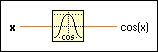# Cosine Function

## LabVIEW 2018 Help

Edition Date: March 2018
Part Number: 371361R-01
View Product InfoLabVIEW 2016 HelpLabVIEW 2017 HelpLabVIEW 2018 HelpLabVIEW 2019 HelpLabVIEW 2020 Help

Owning Palette: Trigonometric Functions

Requires: Base Development System

Computes the cosine of x, where x is in radians.

The connector pane displays the default data types for this polymorphic function.

Examplex can be a scalar number, array or cluster of numbers, array of clusters of numbers, and so on.cos(x) is of the same numeric representation as x. When x is of the form x = a + bi, that is, when x is complex, the following equation defines cos(x): cos(x) = cos(a) * cosh(b) + i(-sin(a) * sinh(b))

## Example

Refer to the Sine VI in the labview\examples\Mathematics\Elementary & Special Functions\Trigonometric Functions directory for an example of using the Cosine function.